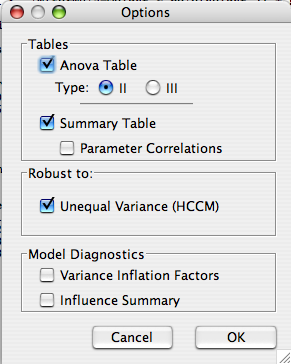## Description

This dialog controls the main tests and diagnostic summaries the model.• Anova Table This is displayed by default, and provides tests of all terms in the model.
• Type Indicates the sum of squares type for the Anova table. Deducer defaults to type II sum of squares because it is arguably the more interpretable type. Many other statistical packages default to type III (e.g. SAS SPSS). Sum of Squares defined.
• Summary table Provides the parameter estimates, standard errors and tests of their significance.
• Parameter correlations Displays the correlation between the model parameters.
• Unequal variance (HCCM) In most linear models there is no a priori reason to expect or assume that the variances are constant across all levels of the predictors, and furthermore, it is difficult to detect departures from equality of variance. As little power is lost by using HCCM even when the variances are constant, it is best to use them unless there is a compelling reason to do otherwise.
• Diagnostics
• Variance inflation factors If the predictors in the model are highly collinear (correlated), this can severely inflate the variances of the parameters, and thus make it difficult to find significant results. As a general rule, inflation factors greater than 5 should be examined closely.
• Influence summary Provides outlier and influence measures for the cases with the highest influence.